Chapter 5

Similar to the electric field, the Gauss Law of Magnetic Field has defined the relationship between magnetic charge and magnetic field. The Gauss Law of Magnetic Field is: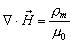(5.1)

In which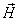is the magnetic field strength,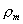is the magnetic charge density.

Combine the Gauss Law of Magnetic Field (5.1) and magnetic charge density distribution equation (3.1), and we get the follows magnetic field strength solution: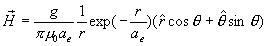(5.2)

We will prove that the above equation (5.2) satisfies the Gauss Law of Magnetic Field.
As we know the gradient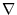in spherical coordinate is: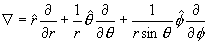From equation (5.2), thus: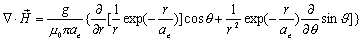(5.3)

Therefore,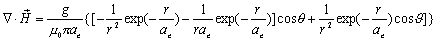(5.4)

Thus: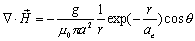(5.5)

Combine equation (3.1) and (5.5), then we get: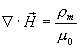Thus we have proved that the magnetic field solution equation (5.2) satisfied the Gauss Law of Magnetic Field.

Reference

W.B.Bonnor

Class.Quant.Grav. 19 (2002) 149-153

Interaction between a stationary electric charge and a stationary magnetic dipole
W.B.Bonnor

Class.Quant.Grav. 18 (2001) 2853